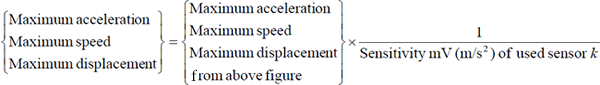Accelerometer & related products FAQThe calibration procedure by using the DS-0021 FFT software when signal is integrated by the PS-1300The PS-1300 calculates the displacement value of 5 V which is mentioned in the instruction manual.

When the sensor sensitivity is [0.9 mV/(m/s2)] and the gain setting of the PS-1300 is ;

Measureable maximum acceleration, speed and displacement

(When the sensor with 1 mV/(m/s2) sensitivity.)

In case of acceleration (ACC m/s2)

 Gain Maximum acceleration(m/s2) 1 2 5 10 20 50 100 5000 2500 1000 500 250 100 50

In case of speed (VEL mm/s)

 Gain Maximum Speed(mm/s) 1 2 5 10 20 50 100 5000 2500 1000 500 250 100 50

In case of displacement (DISP mm)

 Gain Maximum displacement(mm) 0.1 0.2 0.5 1 2 5 10 500 250 100 50 25 10 5

In case of other than the sensor with 1 mV/(m/s2) sensitivity is used, please check with the following formula before use.From the above formula;

V/EU setting value = Sensor sensitivity × 5 ÷ The maximum displacement value in the above formula which can be obtained by the gain setting (sensor sensitivity: the value of mV/(m/s2))

The actual settings for the above example;

Set the unit [mm] and physical quantity [0.9 V/EU] by the input menu [Unit/ Calibration] of the DS-0221. Setting for the speed is the same, but the unit is [mm/s].

In addition, these calibration methods are same for other FFT Analyzers such as CF-7200.

Revised:2007/08/20